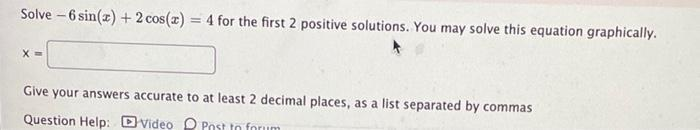Question:

# Solve - 6 sin(x) + 2 cos(x) 4 for the first 2 positive solutions. You may solve this equation graphically. X = Give your answersSolve - 6 sin(x) + 2 cos(x) 4 for the first 2 positive solutions. You may solve this equation graphically. X = Give your answers accurate to at least 2 decimal places, as a list separated by commas Question Help: D Video Post In forum